##### The Riemann Sum!

Let's talk about the Riemann Sum!!

Okay, so the Riemann Sum does the same thing that an integral does, as it is essentially measuring the area under a curve! What this means for 𝛑, is that you can measure the area (well, approximate the are) of a semicircle, multiply it by 2 to get the area of a circle, and divide by r**2, to solve for an approximate value of 𝛑!

To imagine the Riemann Sum properly, think of a semicircle, with both ends touching the x axis. Now, imagine this: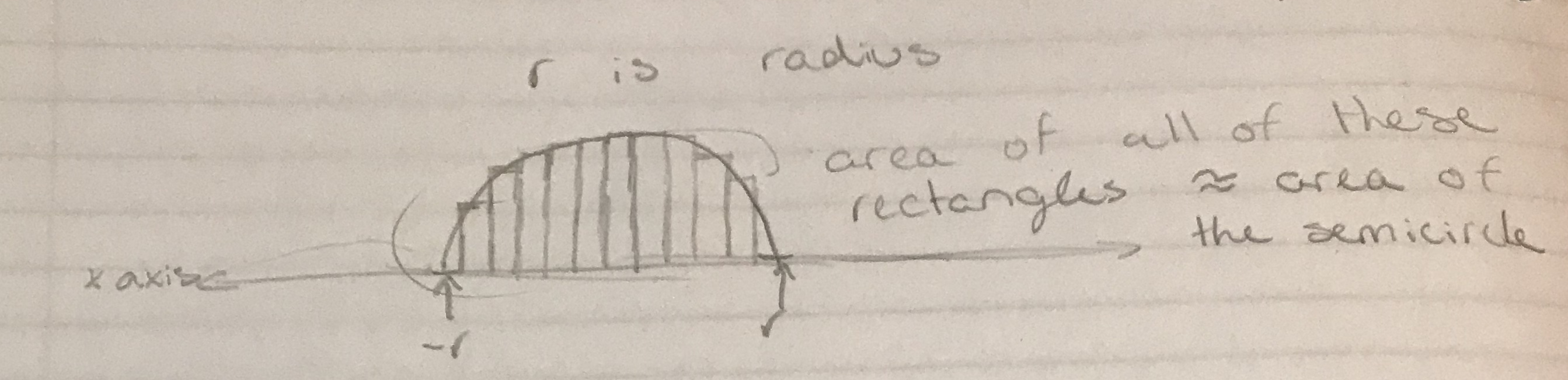This is a good visualization of integration (or more specifically, the Riemann Sum), but what is actually going on?

Well, there are two ways to explain this: The Calculus way, or the Summation way.

First off, the Calculus method is fairly concise and easy to read, but does not really help all that much: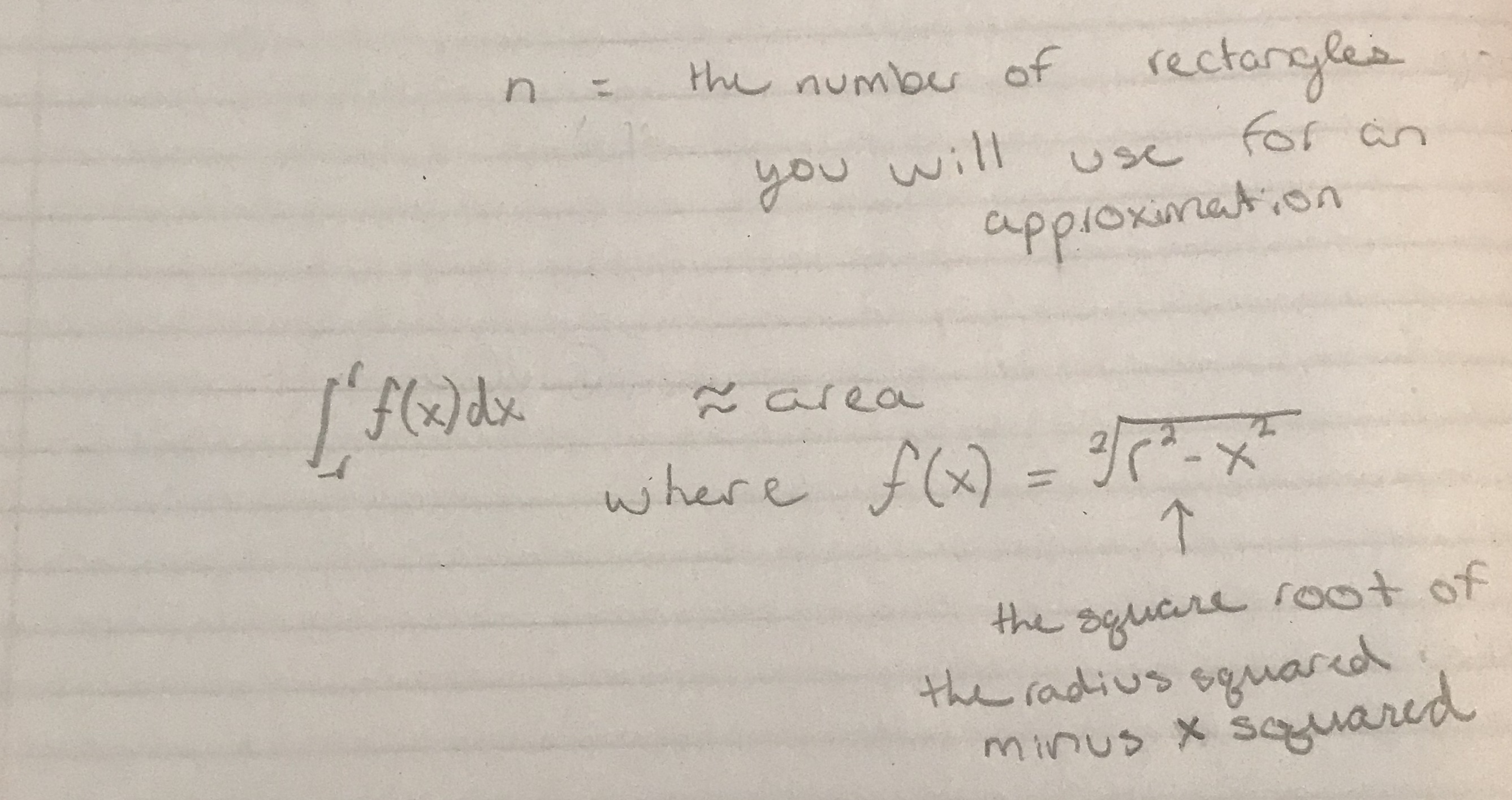Cool, right? (Note from after I took that photo, the integration is not approximately the area, it is exactly the area.)

Now, the Summation way (aka the Riemann Sum) makes all this so much clearer!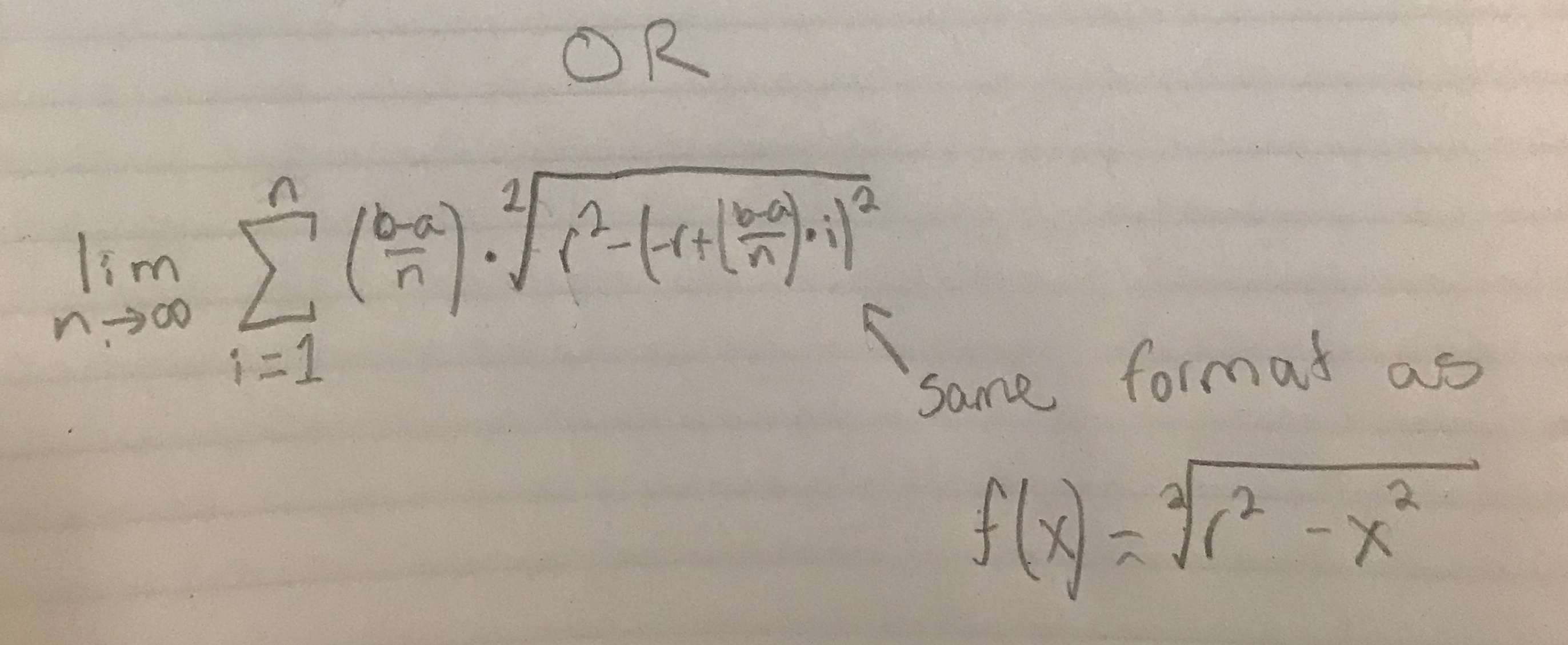(In case you do not know how to read this one, it is basically saying that, as n → ∞, it sums all of the areas of all n rectangles that fit under the curve.)

Note that, when plugged into a calculator, you can say: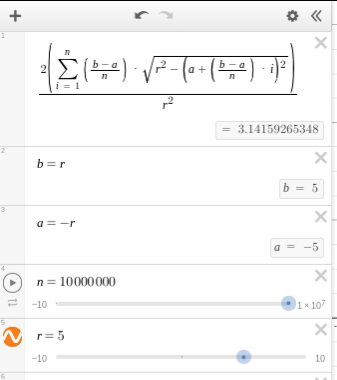Please let me know if you have any further questions!!!
^ ^*

Oh, also, please pardon my poor penmanship.. T ~ T I have not written English too much lately. (Lol)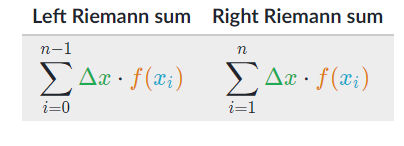You are viewing a single comment. View All
DynamicSquid (4629)

@LizFoster Cool! I'll definitely check them out. Thanks!